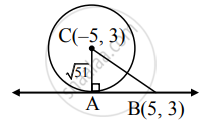# Answer the following : Find the length of the tangent segment drawn from the point (5, 3) to the circle x2 + y2 + 10x – 6y – 17 = 0 - Mathematics and Statistics

Sum

Find the length of the tangent segment drawn from the point (5, 3) to the circle x2 + y2 + 10x – 6y – 17 = 0

#### SolutionGiven equation of circle is

x2 + y2 + 10x – 6y – 17 = 0

Comparing this equation with

x2 + y2 + 2gx + 2fy + c = 0, we get

2g = 10, 2f = –6, c = –17

∴ g = 5, f = –3, c = –17

Centre of circle = (– g, – f )

= C(– 5, 3)

Radius of circle = sqrt("g"^2 + "f"^2 - "c")

= sqrt(5^2 + (-3)^2 - (-17))

= sqrt(25 + 9 + 17)

= sqrt(51)

BC = sqrt((-5 - 5)^2 + (3 - 3)^2

= sqrt(100 + 0)

= 10

In right angled ΔABC

BC2 = AB2 + AC2  …[Pythagoras theorem]

∴ (10)2 = "AB"^2 + (sqrt(51))^2

∴ AB2 = 100 – 51 = 49

∴ AB = 7

∴ Length of the tangent segment from (5, 3) is 7 units.

Alternate method:

Given equation of circle is

x2 + y2 + 10x – 6y – 17 = 0

Here, g = 5, f = –3, c = –17

Length of the tangent segment to the circle

x2 + y2 + 2gx + 2fy + c = 0 from the point

(x1, y1) is sqrt(x_1^2 + y_1^2 + 2"g"x_1 + 2"f"y_1 + "c").

∴ Length of the tangent segment from (5, 3)

= sqrt((5)^2 + (3)^2 + 10(5) - 6(3) - 17)

= sqrt(25 + 9 + 50 - 18 - 17)

=  sqrt(49)

= 7 units

Is there an error in this question or solution?

#### APPEARS IN

Balbharati Mathematics and Statistics 1 (Arts and Science) 11th Standard Maharashtra State Board
Chapter 6 Circle
Miscellaneous Exercise 6 | Q II. (14) | Page 138